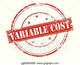# Variable Cost

By Research DeskVariable cost is the cost that varies with respect to production volume or the amount of services provided. This cost can be Nil if there is no production or service.

Variable cost per unit can be calculated using the following formula:

Variable Cost per unit = Total Quantity Produced / Total Variable Cost (e.g. material, labour, taxes etc.)

e.g. If 100 bicycles are produced with tyre cost of Rs. 5,00,000, sheet metal cost of Rs. 1,00,000, electronic components of Rs. 2,00,000 and assembly chain wage bill of Rs. 50,000, total variable cost of the bicycles is Rs. 8,50,000.

The, variable cost per bicycle = 8,50,000 / 100 = Rs. 8,500

The major portion of the variable costs are covered by direct materials. Direct labour can be or cannot be a part of the variable cost depending on whether the amount was varied based on the change in production volumes. Overheads are usually not considered as variable cost.

Variable cost is part of the total cost, other being fixed cost. A company having large proportion of costs as variable costs compared to its fixed costs will vary its expenses in direct proportion with revenues. Thus, it is easy to achieve break even in a company having low variable costs, but the profitability margin may get reduced accordingly.

It is much more easy for analysts to analyse a company with more variable costs as they may show more consistent cost per unit and are therefore more predictable

Some common examples of variable costs are: Raw Materials, Freight, commissions, Labor which is directly associated to production.